Next: Parametric polynomial Up: Simplification procedures Previous: Simplification procedures   Contents

### Deflation

The procedure DeflationUP will create a simplification procedure based on a re-writting of the univariate polynomial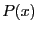of degree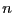. Let us assume that approximate roots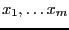of the polynomial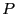has been found. Then the polynomial may be written as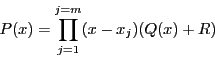where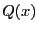has degree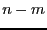. In the generated simplification procedure a C++ program will use the ALIAS_Nb_Solution approximate roots stored in the interval matrix ALIAS_Solution to compute safely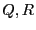and will then use the above form ofto compute the interval evaluation offor the current box. If this evaluation does not include 0, then the simplification procedure will return -1, allowing the current box to be discarded.

The syntax of this procedure is:


DeflationUP(Func,Vars,EvalProc,JevalProc,name)

where
• Func: thepolynomial
• Vars: the name of the polynomial variable
• EvalProc: the name of a C++ procedure in MakeF format that will be used to evaluate the polynomial. If GradientSolve or HessianSolve are used as solving procedure this name is by default "F". Otherwise the user may use its own procedure, for example by using MakeF.
• JevalProc: the name of a C++ procedure in MakeJ format that will be used to evaluate the derivative of the polynomial. If GradientSolve or HessianSolve are used as solving procedure this name is by default "J". Otherwise the user may use its own procedure, for example by using MakeJ.This procedure is used to compute accurately the approximate roots ofby using the Newton scheme with as initial guess the mid-point of the global C++ variable ALIAS_Solution until the residues are lower than ALIAS/fepsilon. Alternatively you may specify "none" for JevalProc in which case the mid-point of ALIAS_Solution will be used as approximate solution
• name: the name of the simplification procedure that will be written in the file name.C
To be used the coefficients ofmust be either real numeric or intervals.Next: Parametric polynomial Up: Simplification procedures Previous: Simplification procedures   Contents
Jean-Pierre Merlet 2012-12-20